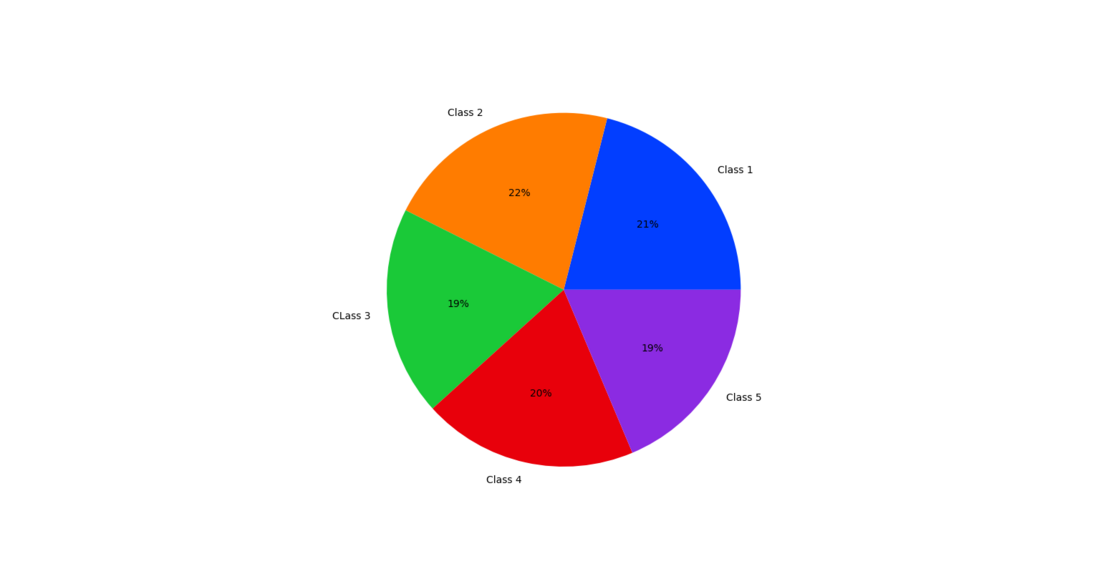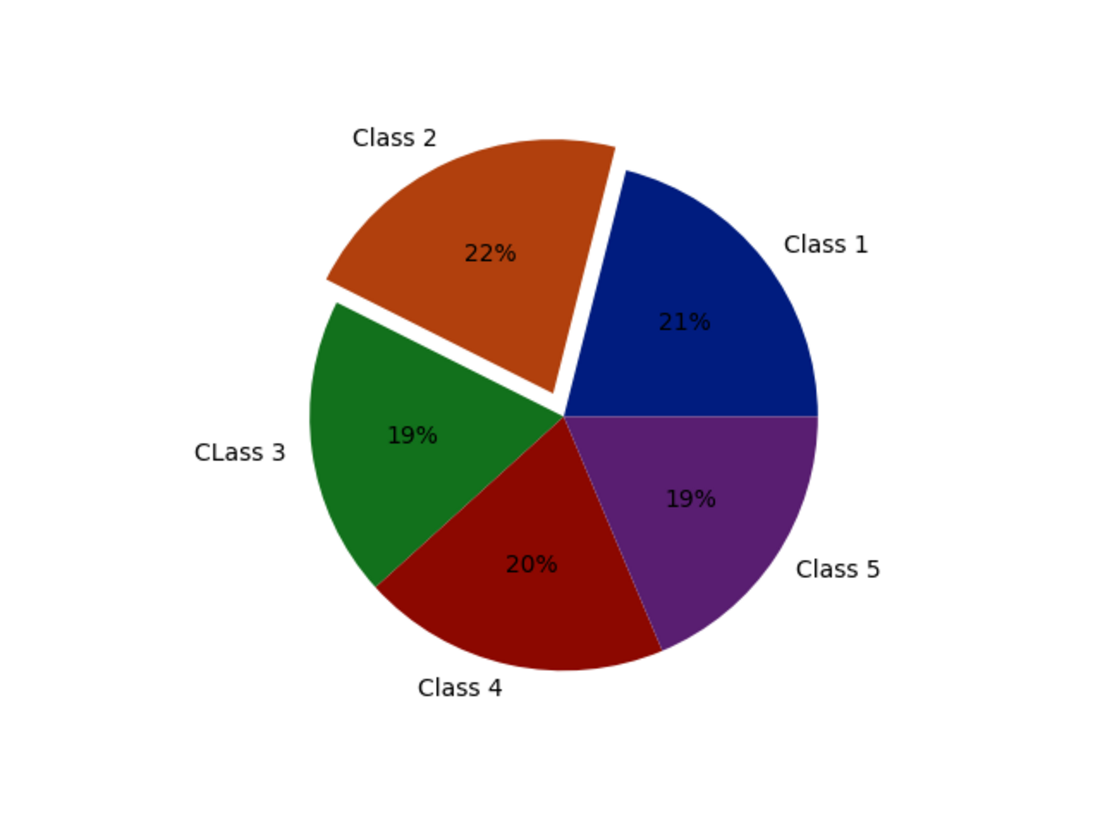Open In App

# How to Create a Pie Chart in Seaborn?

In this article, we will learn how to plot pie charts using seaborn and matplotlib.

Seaborn is a Python data visualization library based on matplotlib. It provides a high-level interface for drawing attractive and informative statistical graphics. Matplotlib is a comprehensive library for creating static, animated, and interactive visualizations in Python. Matplotlib is a multi-platform data visualization library built on NumPy arrays and designed to work with the broader SciPy stack.

Syntax to install seaborn and matplotlib libraries:

pip install seaborn

pip install matplotlib

Example 1:

Let’s take an example of 5 classes with some students in it and plot a pie chart on the basic number of students in each class.

## Python3

 `# importing libraries``import` `matplotlib.pyplot as plt``import` `seaborn`` ` `# declaring data``data ``=` `[``44``, ``45``, ``40``, ``41``, ``39``]``keys ``=` `[``'Class 1'``, ``'Class 2'``, ``'CLass 3'``, ``'Class 4'``, ``'Class 5'``]`` ` `# define Seaborn color palette to use``palette_color ``=` `seaborn.color_palette(``'bright'``)`` ` `# plotting data on chart``plt.pie(data, labels``=``keys, colors``=``palette_color, autopct``=``'%.0f%%'``)`` ` `# displaying chart``plt.show()`

Output:Example 2:

Now let’s plot a pie chart with a different palette and explode.

## Python3

 `# importing libraries``import` `matplotlib.pyplot as plt``import` `seaborn`` ` `# declaring data``data ``=` `[``44``, ``45``, ``40``, ``41``, ``39``]``keys ``=` `[``'Class 1'``, ``'Class 2'``, ``'CLass 3'``, ``'Class 4'``, ``'Class 5'``]`` ` `# declaring exploding pie``explode ``=` `[``0``, ``0.1``, ``0``, ``0``, ``0``]``# define Seaborn color palette to use``palette_color ``=` `seaborn.color_palette(``'dark'``)`` ` `# plotting data on chart``plt.pie(data, labels``=``keys, colors``=``palette_color,``        ``explode``=``explode, autopct``=``'%.0f%%'``)`` ` `# displaying chart``plt.show()`

Output: